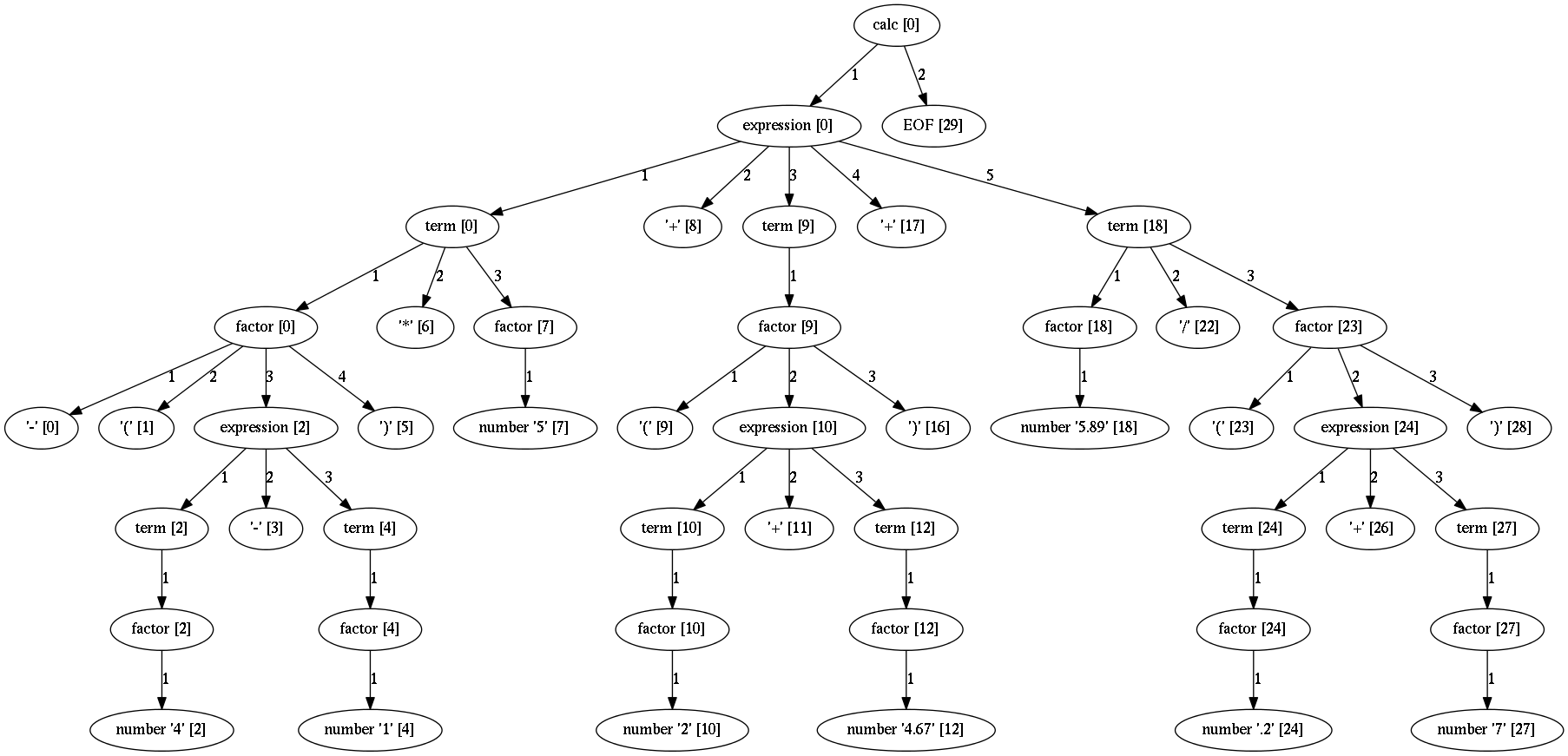# Parse trees¶

Parse tree is a first structure you get from a successful parse.

Parse tree or concrete syntax tree is a tree structure built from the input string during parsing. It represent the structure of the input string. Each node in the parse tree is either a terminal or non-terminal. Terminals are the leafs of the tree while the inner nodes are non-terminals.

Here is an example parse tree for the `calc` grammar and the expression `-(4-1)*5+(2+4.67)+5.89/(.2+7)`:Each non-leaf node is non-terminal. The name in this nodes are the names of the grammar PEG rules that created them.

The leaf nodes are terminals and they are matched by the string match or regex match rules.

In the square brackets is the location in the input stream where the terminal/non-terminal is recognized.

Each parse tree node has the following attributes:

• rule - the parsing expression that created this node.
• rule_name - the name of the rule if it was the root rule or empty string otherwise.
• position - the position in the input stream where this node was recognized.
• position_end - the end of the node in the input stream. This index is one char behind the last char that belongs to this node. Thus, ```position_end - position == length of the node```.

If you want to get line and column from position you can use `pos_to_linecol` parser method.

``````  line, col = parser.pos_to_linecol(node.position)
``````

## Terminal nodes¶

Terminals in Arpeggio are created by the specializations of the parsing expression `Match` class. There are two specialization of `Match` class:

• `StrMatch` if the literal string is matched from the input or
• `RegExMatch` if a regular expression is used to match input.

To get the matched string from the terminal object just convert it to string (e.g. `str(t)` where `t` is of `Terminal` type).

## Non-terminal nodes¶

Non-terminal nodes are non-leaf nodes of the parse tree. They are created by PEG grammar rules. Children of non-terminals can be other non-terminals or terminals.

For example, nodes with the labels `expression`, `factor` and `term` from the above parse tree are non-terminal nodes created by the rules with the same names.

`NonTerminal` inherits from `list`. The elements of `NonTerminal` are its children nodes. So, you can use index access:

``````child = pt_node
``````

Or iteration:

``````for child in pt_node:
...
``````

Additionally, you can access children by the child rule name:

For example:

``````# Grammar
def foo(): return "a", bar, "b", baz, "c", ZeroOrMore(bar)
def bar(): return "bar"
def baz(): return "baz"

# Parsing
parser = ParserPython(foo)
result = parser.parse("a bar b baz c bar bar bar")

# Accessing parse tree nodes. All asserts will pass.
# Index access
assert result.rule_name  == 'bar'
# Access by rule name
assert result.bar.rule_name == 'bar'

# There are 8 children nodes of the root 'result' node.
# Each child is a terminal in this case.
assert len(result) == 8

# There is 4 bar matched from result (at the beginning and from ZeroOrMore)
# Dot access collect all NTs from the given path
assert len(result.bar) == 4
# You could call dot access recursively, e.g. result.bar.baz if the
# rule bar called baz. In that case all bars would be collected from
# the root and for each bar all baz will be collected.

# Verify position
# First 'bar' is at position 2 and second is at position 14
assert result.bar.position == 2
assert result.bar.position == 14

``````

## Printing parse trees¶

Elements of the parse tree has `tree_str` method which can be used to print the tree.

For example:

``````...
parse_tree = parser.parse(some_input)
print(parse_tree.tree_str())
``````

Result might look something like:

``````simpleLanguage=Sequence [0-191]
keyword=Kwd(function) [0-8]: function
symbol=RegExMatch(\w+) [9-12]: fak
parameterlist=Sequence [13-20]
symbol=RegExMatch(\w+) [13-14]: n
symbol=RegExMatch(\w+) [16-17]: x
symbol=RegExMatch(\w+) [19-20]: p
block=Sequence [22-191]
StrMatch({) [22-23]: {
statement=Sequence [28-189]
ifstatement=Sequence [28-188]
keyword=Kwd(if) [28-30]: if
StrMatch(() [31-32]: (
expression=OrderedChoice [32-36]
operation=Sequence [32-36]
symbol=RegExMatch(\w+) [32-33]: n
operator=RegExMatch(\+|\-|\*|\/|\=\=) [33-35]: ==
literal=RegExMatch(\d*\.\d*|\d+|".*?") [35-36]: 0
StrMatch()) [36-37]: )
block=Sequence [38-93]
StrMatch({) [38-39]: {
statement=Sequence [78-87]
returnstatement=Sequence [78-86]
keyword=Kwd(return) [78-84]: return
expression=OrderedChoice [85-86]
literal=RegExMatch(\d*\.\d*|\d+|".*?") [85-86]: 0
StrMatch(;) [86-87]: ;

``````

Each line contains information about the rule and the span of the input where the match occurred.

## Parse tree reduction¶

Parser can be configured to create a reduced parse tree. More information can be found here.

## Suppressing parse tree nodes¶

This feature is available for `ParserPython` only. Rule classes may have a class level `suppress` attribute set to `True`. Parse tree nodes produced by these rules will be removed from the resulting parse tree. This may be handy to reduce syntax noise from the punctuation and such in the resulting parse tree.

``````class SuppressStrMatch(StrMatch):
suppress = True

def grammar():
return "one", "two", SuppressStrMatch("three"), "four"

parser = ParserPython(grammar)

result = parser.parse("one two three four")
assert len(result) == 3
assert result == "two"
assert result == "four"
``````

This feature can nicely be combined with overriding of special rule classes.